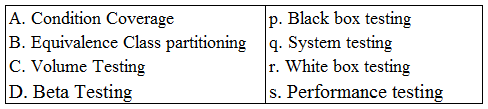# ISRO Scientist or Engineer Computer Science Dec 2017

Instructions

For the following questions answer them individually

Question 71

# A 32 bit adder is formed by cascading 4 bit CLA adder. The gate delays (latency) for getting the sum bits isQuestion 72

# We consider the addition of two 2’s compliment numbers $$b_{n - 1}b_{n - 2}.......b_{0}$$ and $$a_{n - 1}a_{n - 2}.........a_0$$. A binary adder for adding two unsigned binary numbers is used to add two binary numbers. The sum is denoted by $$c_{n - 1}c_{n - 2}.......c_{0}$$ The carry out is denoted by $$c_{out}$$. The overflow condition is identified byQuestion 73

# Consider the functionint fun(x: integer){ If x > 100 then fun = x - 10; else fun = fun(fun(x + 11));}For the input x = 95, the function will returnQuestion 74

# Consider the functionint func(int num) {int count = 0;while(num) { count++; num >>= 1;}return(count) ;}For func(435) the value returned isQuestion 75

# In IEEE floating point representation, the hexadecimal number 0xC0000000 corresponds toQuestion 76

# Which of the following set of components is sufficient to implement any arbitrary Boolean function?Question 77

# Consider the following :Matching A, B, C, D in the same order gives.Question 78

# Consider the results of a medical experiment that aims to predict whether someone is going to develop myopia based on some physical measurements and heredity. In this case, the input data set consists of the person’s medical characteristics and the target variable is binary: 1 for those who are likely to develop myopia and 0 for those who aren’t. This can be best classified asQuestion 79

# Which of the following related to snowflake schema is true?Question 80

# Consider the following C function#include <stdio.h>int main(void){ char c[ ] = "ICRBCSIT17"; char *p=c; printf("%s", c+2[p] - 6[p] - 1); return 0;}The output of the program isOR